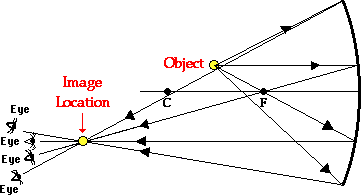Home » Posts tagged 'john abraham'

# Tag Archives: john abraham

## Alternating Current circuits

“a circuit has a battery of emf E and internal resistance r.two inductors of inductance L1 and L2 are connected in series to the battery but parallel to each other.what is the current through each inductor in steady state?”Answer: A pure inductor does not offer any reactance to pure dc. Therefore the only resistance in circuit is the internal resistance. But since the two inductors are in parallel, current through each of them is half the total current in circuit, = E/2r

## Optics MCQ – image formation by concave mirror

I am having trouble with a problem.

Q. In an experiment to determine the focal length (f) of a concave mirror by the u–v method, a student places the object pin A on the principal axis at a distance x from the pole P. The student looks at the pin and its inverted image from a
distance keeping his/her eye in line with PA. When the student shifts his/her eye towards left, the image appears to the right of the object pin. Then

(A) x < f

(B) f < x < 2f

(C) x = 2f

(D) x > 2fEXPLANATION

The image and the object will move together at 2f

The one closer to the eye will move faster

In the question, the situation occurs when the image is closer to the eye and this happens when the object is between f and 2f